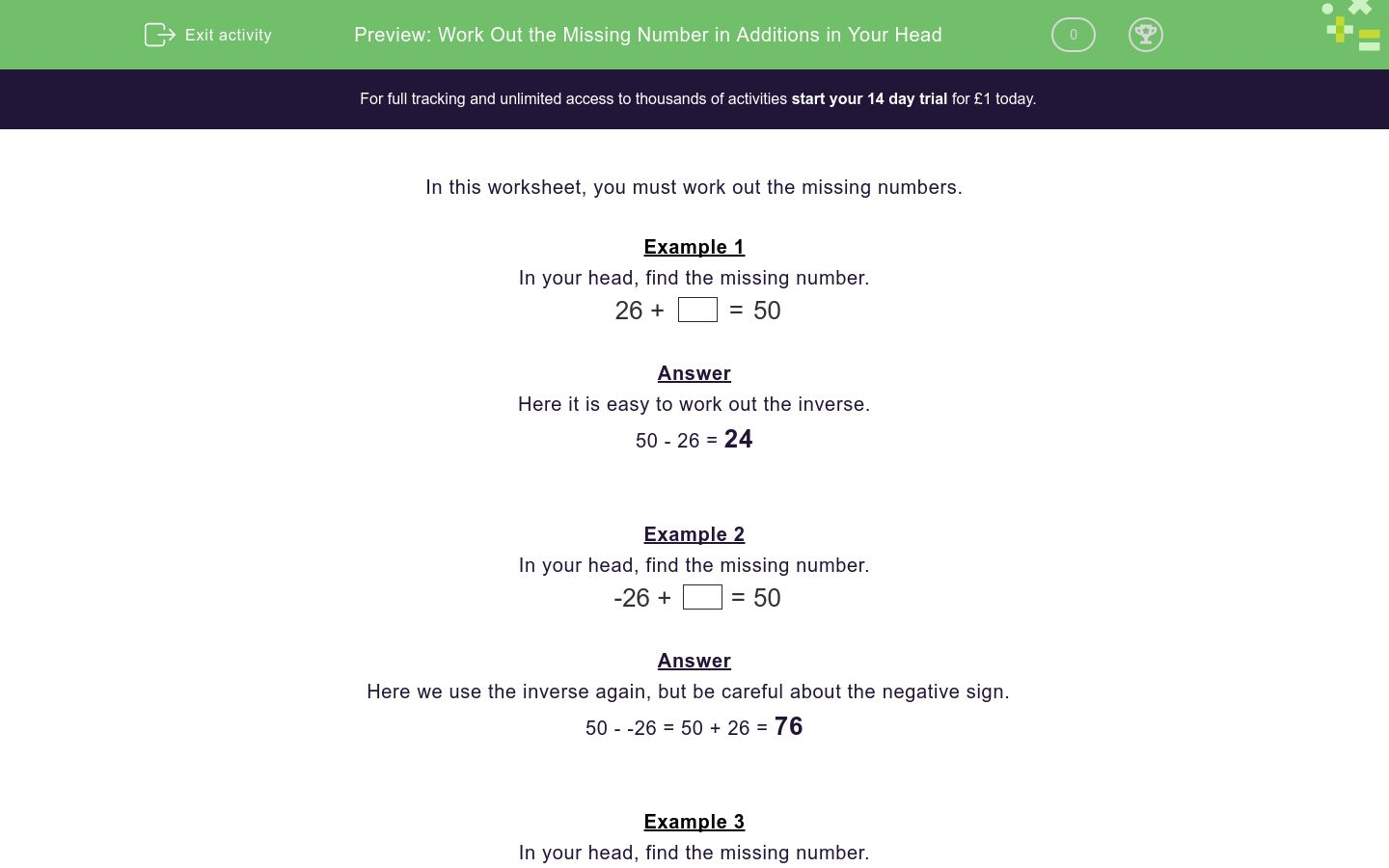### Affordable pricing from £10/month

In this worksheet, students must work out the missing number in addition sums.Key stage:  KS 2

Curriculum topic:   Number: Addition and Subtraction

Difficulty level:### QUESTION 1 of 10

In this worksheet, you must work out the missing numbers.

Example 1

26 + = 50

Here it is easy to work out the inverse.

50 - 26 = 24

Example 2

-26 + = 50

Here we use the inverse again, but be careful about the negative sign.

50 - -26 = 50 + 26 = 76

Example 3

0.81 + = 1

Here it is easy to work out the inverse.

1 - 0.81 = 1.00 - 0.81 = 0.19

It may help to think of 100 - 81 = 19.

20 + = 55

0.2 + = 1

31 + = 100

0.6 + = 1

-27 + = 100

0.26 + = 1

59 + = 100

0.3 + = 1

0.67 + = 1

-20 + = 50
• Question 1

20 + = 55
35
EDDIE SAYS
55 - 20 = 35
• Question 2

0.2 + = 1
0.8
EDDIE SAYS
1 - 0.2 = 0.8
• Question 3

31 + = 100
69
EDDIE SAYS
100 - 31 = 69
• Question 4

0.6 + = 1
0.4
EDDIE SAYS
1 - 0.6 = 0.4
• Question 5

-27 + = 100
127
EDDIE SAYS
100 - -27 = 100 + 27 = 127
• Question 6

0.26 + = 1
0.74
EDDIE SAYS
1 - 0.26 = 0.74
• Question 7

59 + = 100
41
EDDIE SAYS
100 - 59 = 41
• Question 8

0.3 + = 1
0.7
EDDIE SAYS
1 - 0.3 = 0.7
• Question 9

0.67 + = 1
0.33
EDDIE SAYS
1 - 0.67 = 0.33
• Question 10

-20 + = 50
70
EDDIE SAYS
50 - -20 = 50 + 20 = 70
---- OR ----

Sign up for a £1 trial so you can track and measure your child's progress on this activity.

### What is EdPlace?

We're your National Curriculum aligned online education content provider helping each child succeed in English, maths and science from year 1 to GCSE. With an EdPlace account you’ll be able to track and measure progress, helping each child achieve their best. We build confidence and attainment by personalising each child’s learning at a level that suits them.

Get started